Power of a QuotientCustom SearchPOWER OF A QUOTIENT The law of exponents for a power of an indicated quotient may be developed from the following example :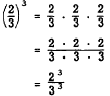Therefore,The law is stated as follows: The power of a quotient is equal to the quotient obtained when the dividend and divisor are each raised to the indicated power separately, before the division is performed. Practice problems. Raise each of the following expressions to the indicated power: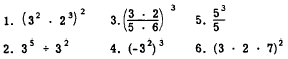Answers: 1. 34 x 26 = 5,184 2. 27 3. 1/125 4. [(-3)2]3 = 729 5. 25 6. 9 x 4 x 49 = 1,764 SPECIAL EXPONENTS Thus far in this discussion of exponents, the emphasis has been on exponents which are positive integers. There are two types of exponents which are not positive integers, and two which are treated as special cases even though they may be considered as positive integers. ZERO AS AN EXPONENT Zero occurs as an exponent in the answer to a problem such as 43 + 43. The law of exponents for division states that the exponents are to be subtracted. This is illustrated as follows: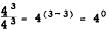Another way of expressing the result of dividing 43 by 43 is to use the fundamental axiom which states that any number divided by itself is 1. In order for the laws of exponents to hold true in all cases, this must also be true when any number raised to a power is divided by itself. Thus, 43/43 must equal 1. Since 43/43 has been shown to be equal to both 40 and 1, we are forced to the conclusion that 40 = 1.  By the same reasoning,Also, 5/5 = 1 Therefore, 50 = 1 Thus we see that any number divided by itself results in a 0 exponent and has a value of 1. By definition then, any number (other than zero) raised to the zero power equals 1. This is further illustrated in the following examples: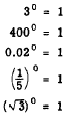ONE AS AN EXPONENT The number 1 arises as an exponent sometimes as a result of division. In the example 53/52 we subtract the exponents to get 53-2= 51 This problem may be worked another way as follows: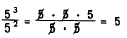Therefore, 51 = 5 We conclude that any number raised to the first power is the number itself. The exponent 1 usually is not written but is understood to exist. NEGATIVE EXPONENTS If the law of exponents for division is extended to include cases where the exponent of the denominator is larger, negative exponents arise. Thus,Another way of expressing this problem Is as follows:Therefore,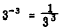We conclude that a number N with a negative exponent is equivalent to a fraction having the following form: Its numerator is 1; its denominator is N with a positive exponent whose absolute value is the same as the absolute value of the original exponent. In symbols, this rule may be stated as follows: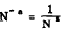Also,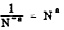The following examples further illustrate the rule: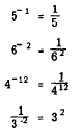Notice that the sign of an exponent may be changed by merely moving the expression which contains the exponent to the other position in the fraction. The sign of the exponent is changed as this move is made. For example,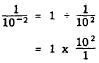Therefore,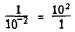By using the foregoing relationship, a problem such as 3+5-4 may be simplified as follows:Integrated Publishing, Inc. - A (SDVOSB) Service Disabled Veteran Owned Small Business